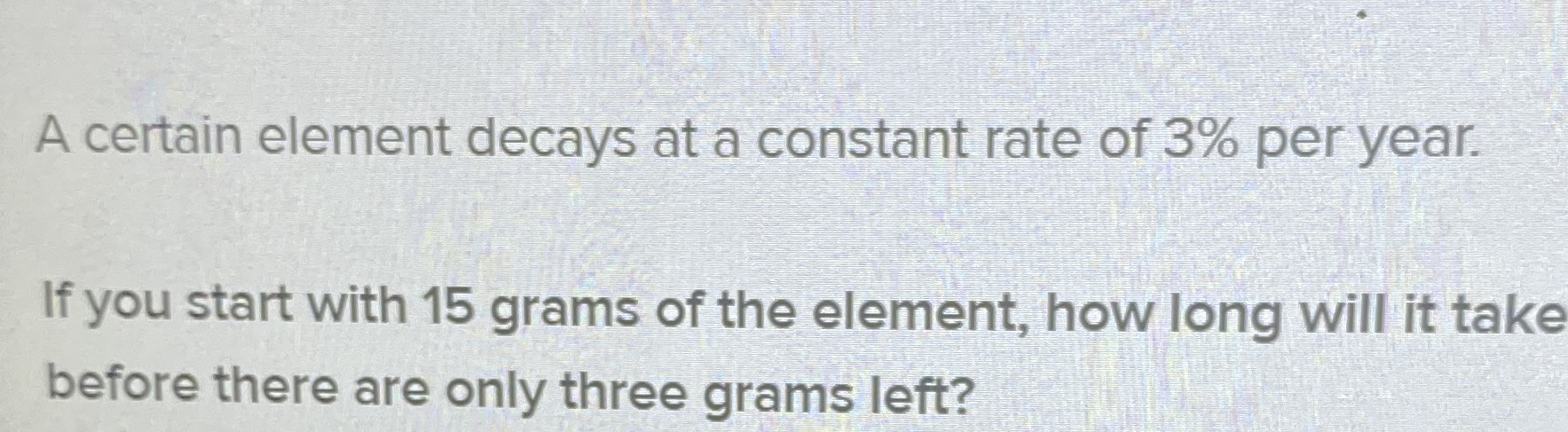### ¿Todavía tienes preguntas de matemáticas?

Pregunte a nuestros tutores expertos
Algebra
PreguntaA certain element decays at a constant rate of $$3 \%$$ per year. If you start with $$15$$ grams of the element, how long will it take before there are only three grams left?

15(1-0.03)$$^{n}$$=3
n$$\approx 53$$